# C++ Queue: The Complete Guide

Queues are an abstract data structure that follows the FIFO algorithm, i.e., the data item put in the queue will be accessed first. Queues are open at both ends, unlike stacks at only one end.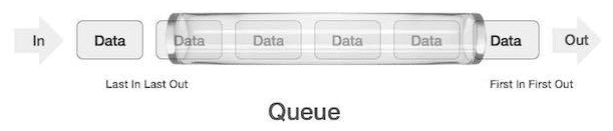## C++ Queue

Queue in C++ is the container adaptor specifically designed to operate in a FIFO context (first-in-first-out), where elements are inserted into one end of the container and extracted from the other.

Queues are implemented as container adaptors, which are classes that use the encapsulated object of the container class as its underlying container, providing the particular set of member functions to access its items.

Elements are pushed into a “back” of the specific container and popped from its “front”. So, for example, we can create a queue in C++ with the following syntax.

`queue<data_type>queue_name`

For example   queue<int>queue1;

Elements in the queue are inserted at the back end and are deleted from the front end.

In STL, there are many functions supported by queues. Some of them are listed below.

The functions supported by the queue are the following.

### empty()

An empty function is used to check whether the queue is empty.

Syntax:

`queue_name.empty()`

An empty() function returns true if the queue is empty or false if the list is not empty.

Note:  There is no parameter passed in the empty function. If we give any parameter, it will show an error.

### size()

The size() function is used to find the queue size.

Syntax:

`queue_name.size()`

The size() function returns several elements present in the queue.

Note: There is no parameter passed in the size function. If we give any parameter, it will show an error.

### swap()

The swap() function is used to swap the elements of two different queues of the same type.

Syntax:

`queue_name1.swap(queue_name2)`

There is no return value. The operation swaps the elements of queue_name1 to queue_name2.

Note: It is essential to have the queues of the same type length of the queues can be variable.

### emplace()

Emplace function is used to insert a new element in the queue.

Syntax:

`queue_name.emplace(value)`

The value parameter holds the value of the element which we want to add to the queue.

The element is added at the back end of the queue.

Note: It is important to have the value parameter of the same type of elements already present in the queue.

### front()

The front function accesses the oldest element in the queue or the element at the front end. As queues follow, first come, first out, it accesses the first element.

Syntax:

`queue_name.front()`

There is no parameter in the front function.

Note: If the queue is empty, the front function shows an undefined behavior.

### back()

The back function fetches the first element from the back end of the later insertion in the queue.

Syntax

`queue_name.back()`

There is no parameter in the back function.

Note: If the queue is empty, the front function shows an undefined behavior.

### push()

The push() function inserts an item in the queue from the back end.

Syntax:

`queue_name.push(value)`

The parameter in the push function is the value we want to push in the queue.

Note: It is important to have the same type of value as the already existing elements.

### pop()

The pop function is used to remove an element from the queue.

Syntax:

`queue.name.pop()`

It removes the oldest element present in the queue of the front element of the queue.

Note: It will show an error if the parameter is passed in the pop function.

## Examples of queues in STL

Write a program to insert three elements in a queue using emplace function and check whether the queue is empty. Then, print a string to show if the queue is empty or not.

```#include <iostream>
#include <queue>
using namespace std;
int main()
{
queue<int> queue1;
queue1.emplace(1);
queue1.emplace(2);
queue1.emplace(3);

if (queue1.empty())
{
cout << "The queue is empty";
}
else
{
cout << "The queue is not empty";
}
return 0;
}```

See the following output.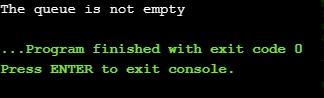Example2: Write a program to add five elements in the queue using the push() function, remove one element using the pop() function, and print the queue afterward.

```#include <iostream>
#include <queue>
using namespace std;

int main()
{
queue<int> queue2;
queue2.push(0);
queue2.push(1);
queue2.push(2);
queue2.push(3);
queue2.push(4);
// queue has element 0,1,2,3,4

queue2.pop();

// after poping one element queue becomes 0,1,2,3

// Printing queue elements
cout << "Elements of the queue are:";
while (!queue2.empty())
{
cout << ' ' << queue2.front();
queue2.pop();
}
}```

See the following output.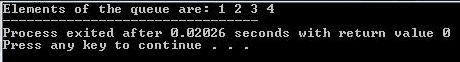Example3: Write a program to print the size of the queue, the front element of the queue, and the back element of the queue.

```#include <iostream>
#include <queue>
using namespace std;

int main()
{
queue<int> queue3;
queue3.push(0);
queue3.push(1);
queue3.push(2);
queue3.push(3);
queue3.push(4);

cout<<"Size of the queue is: "<<queue3.size()<<endl;
cout<<"The element at the front is:"<<queue3.front()<<endl;
cout<<"The element at the back is:"<<queue3.back()<<endl;
return(0);
}
```

See the following output.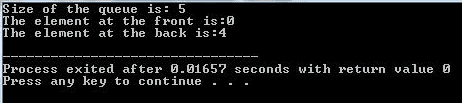Example4: Create two queues, add elements to both queues, swap the queues, and print the swapped queues.

```#include <iostream>
#include <queue>
using namespace std;

int main()
{
queue<int> queue2;
queue<int> queue1;
queue1.push(0);
queue1.push(1);
queue1.push(2);
queue1.push(3);
queue1.push(4);

queue2.push(5);
queue2.push(6);
queue2.push(7);
queue2.push(8);
queue2.push(9);

queue1.swap(queue2); //Swapping

cout << "Elements of queue1 after swapping are:";
while (!queue1.empty())
{
cout << queue1.front() << " ";
queue1.pop();
}

// Print the second set
cout << endl
<< "Elements of queue2 after swapping are:";
while (!queue2.empty())
{
cout << queue2.front() << " ";
queue2.pop();
}

return 0;
}```

See the output.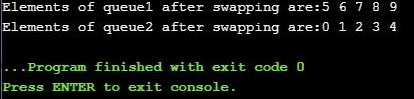That’s it for this tutorial.

Categories C++

This site uses Akismet to reduce spam. Learn how your comment data is processed.